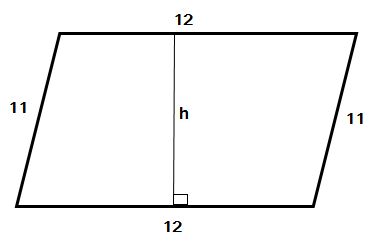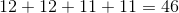# SSAT Middle Level Math : How to find the perimeter of a parallelogram

## Example Questions

### Example Question #1 : How To Find The Perimeter Of A Parallelogram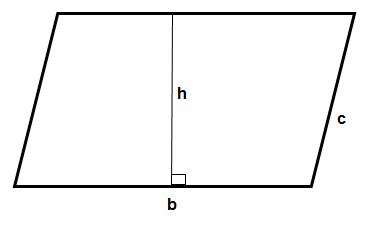Find the perimeter of the given parallelogram if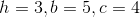14

16

18

12

18

Explanation:

In order to find the perimeter, we find the sum of the OUTER sides: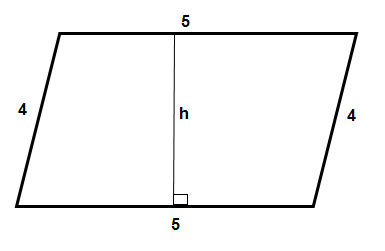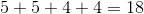Notice that we didn't use the height in our calculation.

### Example Question #2 : How To Find The Perimeter Of A ParallelogramFind the perimeter of the given parallelogram if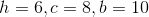22

36

24

38

36

Explanation:

In order to find the perimeter of the parallelogram, we must add the OUTER edges: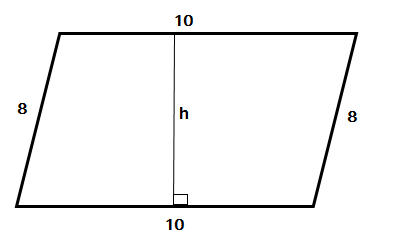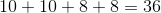Notice that we didn't use height in our calculation.

### Example Question #101 : QuadrilateralsFind the perimeter of the given parallelogram if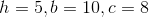23

36

30

26

36

Explanation:

In order to find the perimeter, we need to find the sum of the outer edges: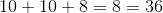Notice that we didn't use height in our calculation.

### Example Question #4 : How To Find The Perimeter Of A Parallelogram

Find the area of a parallelogram with base length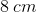and side length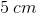.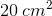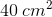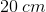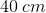Not enough information provided

Not enough information provided

Explanation:

The areaof a parallelogram with heightand basecan be found with the equation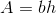. However, while we have been given the length of the base and of another side of the parallelogram, we still do not know the length of the base. Therefore, we do not have enough information to find the area.

### Example Question #5 : How To Find The Perimeter Of A Parallelogram

Find the perimeter of a parallelogram with a height of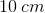, a base of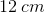, and a side of length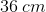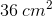Explanation:

The perimeter of a parallelogram is the sum of the length of its sides. Since we know the length of the base and of another side, we know that the remaining sides of the parallelogram match those lengths. Therefore: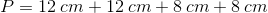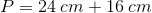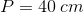### Example Question #141 : Plane GeometryFind the perimeter of the parallelogram if h=, b=, and c=.Explanation:

In order to find the perimeter, find the sum of each OUTER side: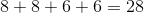Notice the height isn't used in this calculation.

Keep in mind that the sides directly opposite of each other are of the same length. This is why we have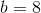and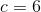appearing twice in our work.

### Example Question #7 : How To Find The Perimeter Of A ParallelogramFind the perimeter of the given parallelogram if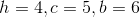Explanation:

In order to find the perimeter, we must find the sum of the outer edges: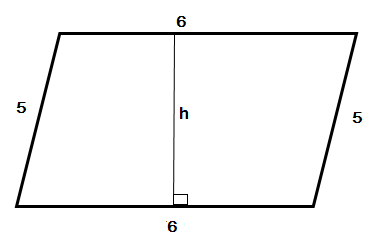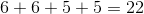Notice that we didn't use height in our calculation.

### Example Question #8 : How To Find The Perimeter Of A ParallelogramFind the perimeter of the given parallelogram if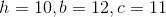In order to find the perimeter, we must find the sum of the outer edges: